• ## mice

2019-09-28 16:15:49
Description 有一天Masha回到家,发现有n只老鼠在它公寓的走廊上,她大声呼叫,所以老鼠们都跑进了走廊的 洞中。 这个走廊可以用一个数轴来表示,上面有n只老鼠和m个老鼠洞。第i只老鼠有一个坐标x i ,第j个洞有 ...

Description
有一天Masha回到家,发现有n只老鼠在它公寓的走廊上,她大声呼叫,所以老鼠们都跑进了走廊的
洞中。
这个走廊可以用一个数轴来表示,上面有n只老鼠和m个老鼠洞。第i只老鼠有一个坐标x i ,第j个洞有
一个坐标p j 和容量c j 。容量表示最多能容纳的老鼠数量。
找 到 让 老 鼠 们 全 部 都 进 洞 的 方 式,使 得 所 有 老 鼠 运 动 距 离 总 和 最 小。 老 鼠i进 入 洞j的 运 动 距 离
为|x i − p j |
无解输出-1。
solution
正解：调整贪心
还是老套路，在能使得答案更小时，我们就直接决策，并加入后悔操作，在这个题目中，我们将老鼠和老鼠洞排个序，然后扫描，开两个堆：老鼠堆和老鼠洞堆.
如果碰到老鼠，我们直接把他丢进最近的一个洞中，计算答案 $$x[i]-p[j]$$ 并且将 $$x[i]+x[i]-p[j]$$ 丢入老鼠堆中.
如果碰到老鼠洞，我们一直把前面能够使得答案更小的老鼠都加入到这个洞中，并且把后悔操作丢入老鼠洞的堆中.
巧妙的是：处理限制的方法：我们在用掉一次老鼠洞后，我们判断是否有剩余容量，如果有，我们再加入一次堆即可
#include <algorithm>
#include <iostream>
#include <cstdlib>
#include <cstring>
#include <cstdio>
#include <cmath>
#include <queue>
#define RG register
using namespace std;
typedef long long ll;
const int N=2000005;
const ll inf=2e15;
inline int gi(){
RG int str=0;RG char ch=getchar();bool h=0;
while(ch!='-' && (ch>'9' || ch<'0'))ch=getchar();
if(ch=='-')h=1,ch=getchar();
while(ch>='0' && ch<='9')str=(str<<1)+(str<<3)+ch-48,ch=getchar();
return h?-str:str;
}

#define x first
#define y second

int n,m;
struct node{
ll x;int y;
node(){}
node(ll _x,int _y){x=_x;y=_y;}
bool operator <(const node &pr)const{return x<pr.x;}
};
pair<ll,int>p[N];
priority_queue<ll>qm;
priority_queue<node>qd;
void work()
{
int tot=0,x,y,j;ll sum=0;
cin>>n>>m;
for(int i=1;i<=n;i++)p[++tot]=make_pair(gi(),-1);
for(int i=1;i<=m;i++){
x=gi();y=gi();sum+=y;
p[++tot]=make_pair(x,y);
}
if(sum<n){puts("-1");return ;}
sort(p+1,p+tot+1);
ll w,ans=0;
for(RG int i=1;i<=tot;i++){
if(p[i].y==-1){
w=inf;
if(!qd.empty()){
w=p[i].x-qd.top().x;j=qd.top().y;qd.pop();
if(p[j].y)p[j].y--,qd.push(node(p[j].x,j));
}
ans+=w;
qm.push(p[i].x+w);
}
else{
while(!qm.empty() && p[i].y && p[i].x-qm.top()<0){
w=p[i].x-qm.top();
p[i].y--;qm.pop();
qd.push(node(p[i].x+w,0));
ans+=w;
}
if(p[i].y)p[i].y--,qd.push(node(p[i].x,i));
}
}
cout<<ans<<endl;
}

int main()
{
work();
return 0;
}


转载于:https://www.cnblogs.com/Hxymmm/p/7795738.html
展开全文• the tidy method performs the <code>mice::pool()</code> portion AND the <code>mice::tidy()</code> part. The reason for this is that the pooling function removes the model objects, and returns a tibble...
• <div><p>Some mice such as the MX Master support connecting over Bluetooth, which works really well and reduces the number of receivers one needs to buy. <p>Unfortunately, Solaar does not support this ...
• from fancyimpute import MICE imputer = MICE() imputed = imputer.complete(dummied) </code></pre> <p>The code crashed with the following stack trace: <pre><code> imputed = imputer.complete...
•HTML
• If the number of the living mice from the previous month is less than or equal to 100 pairs, the new born mice of this month will stay in this lab, if the number of living mice exceeds 100 pairs, the...as each it less
• Mice是一个语义化的前端框架。安装使用：添加这行代码到你应用的 Gemfile：gem 'mice'或gem 'mice', :git => 'git@github.com:miclle/mice.git'然后运行bundle install或自行安装为：\$ gem install mice ...开源项目
• If the number of the living mice from the previous month is less than or equal to 100 pairs, the new born mice of this month will stay in this lab, if the number of living mice exceeds 100 pairs, the...Golang erlang r语言
• <div><p>libratbag should be taught to talk to Razer mice. <p>Razer uses proprietaty USB messages to perform device configuration. An implementation of some Razer mouse protocols can be found in ...
• When I turn the mice on/off they popup in Device Manager as HID-compliant mouse, but don't work, and are not shown in Monitor.ahk. </p><p>该提问来源于开源项目：evilC/AutoHotInterception</p>...
•JavaScript
• 链式方程的多重插补（MICE，Multiple Imputation by Chained Equations）通过一系列迭代的预测模型来“填充”（插补）数据集中的缺失数据。在每次迭代中，将使用数据集中的其他变量来估算数据集中的每个指定的变量，...
原文：miceforest: Fast Imputation with Random Forests in Python
链式方程的多重插补（MICE，Multiple Imputation by Chained Equations）通过一系列迭代的预测模型来“填充”（插补）数据集中的缺失数据。在每次迭代中，将使用数据集中的其他变量来估算数据集中的每个指定的变量，这些迭代持续运行，直到满足收敛为止。
一，MICE的算法实现
MICE的算法如下图所示，这个过程会持续执行，直到所有指定的变量都被插补为止。如果出现插补的均值没有收敛的情况，那么将会执行更多的迭代，尽管通常不需要超过5次迭代。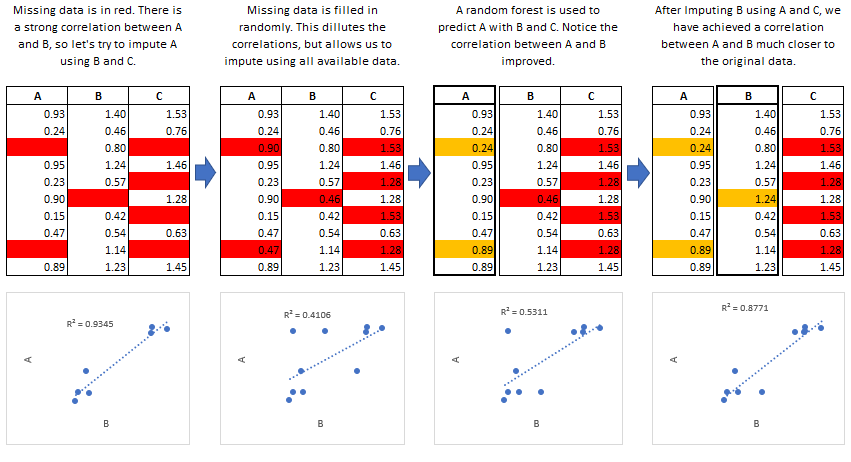MICE适用的场景：
数据泄露(Data Leakage) ：如果缺失值与目标变量直接相关联，从而导致泄漏，则MICE尤其有用。 例如，假设您要对客户保留率进行建模，在客户注册时或注册后1个月通过特定变量手机客户的登录信息，如果该变量的值缺失，这将导致数据泄漏，因为它告诉您“客户留存的时间没有超过1个月”。

Data Leakage定义：
存在和利用这种倒‘因’为‘果’的feature的现象，叫数据竞赛中的Data Leakage。 这里的Data Leakage 跟其他场合说的数据安全数据泄漏完全不一样。从字面上理解，我们说的Data Leakage不是数据泄漏，而是因果关系的纰漏，是由于数据准备过程中出现的失误，使模型沿着有纰漏的，甚至是颠倒的因果关系进行预测，但得到极好的预测结果。
举个例子，Chris老师在处理电信用户流失的时候，用原有的数据集轻轻松松就可以把AUC达到0.99以上。这让人非常警惕。于是Chris老师仔细查看了一下模型和数据，原来数据中有一个权重极高的feature是“3个月内的缴费纪录”。很多流失用户的账户内，这个feature的值是0。再进一步，他跟会计核实了一下，在会计记账中，这个feature 代表的是用户已经流失后的三个月的缴费纪录，那肯定就是0了。这是典型的因果关系颠倒。

漏斗分析（Funnel Analysis）：信息通常是在“漏斗”的不同阶段收集的，MICE可用于对漏斗中不同阶段的实体特征进行有根据的猜测。
置信区间（Confidence Intervals）：MICE可用于插补（估算）缺失值，但是请务必记住，这些估算值是一种预测，创建具有不同估算值的多个数据集可以执行两种类型的推断：

估算值分布：可以为每个估算值构建一个profile（画像，或特征），以获得该值可能的分布信息。
模型预测分布：使用多个数据集，您可以构建多个模型，并为每个样本创建预测的分布。那些具有估算值的样本，如果没有高置信度（插补值没有高置信区间），那么它们的预测值将具有较大的方差，很有可能具有很大的偏差。

二，预测均值匹配
miceforest可以利用称为预测均值匹配（predictive mean matching，PMM）的过程来选择要估算的值。 PMM包含从原始的、完整的数据中选择一个数据点，该数据点的预测值接近缺失样本的预测值。选择最接近的N个（mean_match_candidates参数）值作为候选值，从候选值中随机选择一个值，这个过程可以逐列指定。
MICE在实践中的工作原理如下图所示：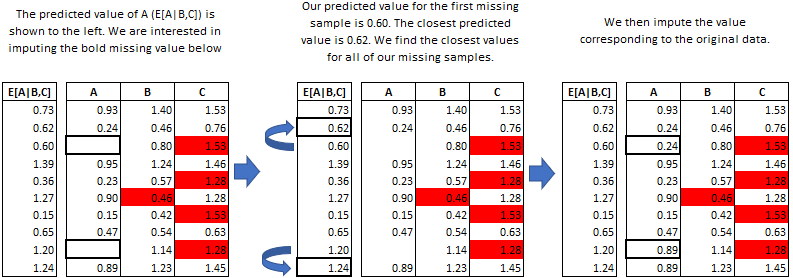对于一个需要插补（估算）的变量，如果该变量具有以下任意特征，那么此方法非常有用：

Multimodal（多模态）
Integer（整数值）
Skewed（偏斜）
从下图中查看这三个特性的特点：

randst = np.random.RandomState(1991)
# random uniform variable
nrws = 1000
uniform_vec = randst.uniform(size=nrws)

def make_bimodal(mean1,mean2,size):
bimodal_1 = randst.normal(size=nrws, loc=mean1)
bimodal_2 = randst.normal(size=nrws, loc=mean2)
bimdvec = []
for i in range(size):
bimdvec.append(randst.choice([bimodal_1[i], bimodal_2[i]]))
return np.array(bimdvec)

# Make 2 Bimodal Variables
close_bimodal_vec = make_bimodal(2,-2,nrws)
far_bimodal_vec = make_bimodal(3,-3,nrws)

# Highly skewed variable correlated with Uniform_Variable
skewed_vec = np.exp(uniform_vec*randst.uniform(size=nrws)*3) + randst.uniform(size=nrws)*3

# Integer variable correlated with Close_Bimodal_Variable and Uniform_Variable
integer_vec = np.round(uniform_vec + close_bimodal_vec/3 + randst.uniform(size=nrws)*2)

# Make a DataFrame
dat = pd.DataFrame(
{
'uniform_var':uniform_vec,
'close_bimodal_var':close_bimodal_vec,
'far_bimodal_var':far_bimodal_vec,
'skewed_var':skewed_vec,
'integer_var':integer_vec
}
)

# Plot the original data
import seaborn as sns
import matplotlib.pyplot as plt
g = sns.PairGrid(dat)
g.map(plt.scatter,s=5)

二模态数据倾向于数据分为两个簇，偏斜分布说明数据的分布不是均匀的。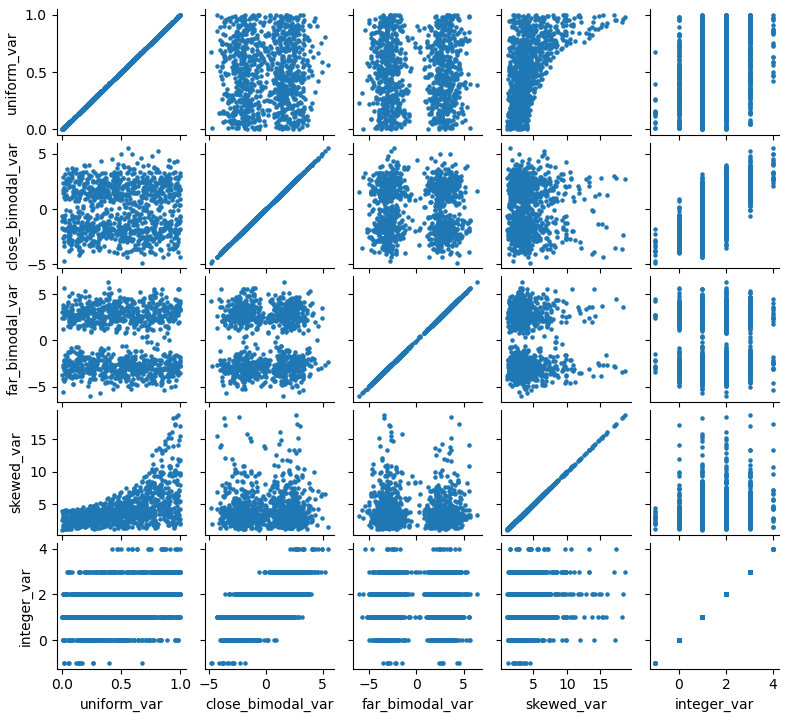三，均值匹配的作用
上图中显示了变量的分布和相关性，让我们运行两次插补过程，一次使用均值匹配，一次使用模型预测，通过比较两次插补的结果来比较均值匹配的效果。
# Ampute the data.
ampdat = mf.ampute_data(dat,perc=0.25,random_state=randst)

# Create kernel
kernelmeanmatch <- mf.MultipleImputedKernel(ampdat,mean_match_candidates=5)
kernelmodeloutput <- mf.MultipleImputedKernel(ampdat,mean_match_candidates=0)

kernelmeanmatch.mice(5)
kernelmodeloutput.mice(5)

案例1，使用均值匹配
kernelmeanmatch.plot_imputed_distributions(wspace=0.2,hspace=0.4)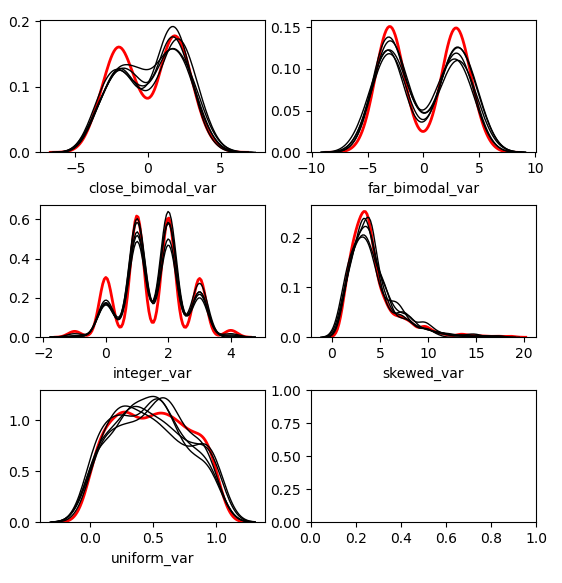案例2，不适用均值匹配
kernelmodeloutput.plot_imputed_distributions(wspace=0.2,hspace=0.4)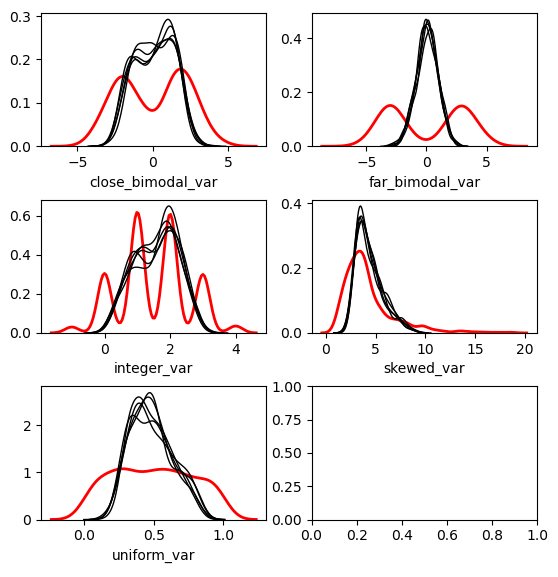注：红线是原始数据，黑线是每个数据集的插补（估算）值。

从案例1和2中，可以看到均值匹配的效果更好，具体情况取决于数据的分布。从直观上来看，简单地从模型预测中返回值，虽然可能会提供更好的“拟合”，但是并不能为插补值提供跟原始值相似的分布。这可能是有益的，具体取决于您的目标。


展开全文机器学习
• t know much about the code, but I assume these mice share some similarities, so maybe some shared code for those mice could be utilized? For this reason, I'm closing my previous #430 issue about ...
• Lougu2295 MICE 给一个 $$n\times m$$ 的矩阵 $$a$$ ，求一条从 $$(1,\ 1)$$ 到 $$(n,\ m)$$ 的最短路径，使得与路径相接的所有网格的权值和最小 $$n,\ m\leq10^3,\ 0\leq a_{i,j}\leq100$$ dp 令 $$f_{0/1,\ i,\... Lougu2295 MICE 给一个 \(n\times m$$ 的矩阵 $$a$$ ，求一条从 $$(1,\ 1)$$ 到 $$(n,\ m)$$ 的最短路径，使得与路径相接的所有网格的权值和最小
$$n,\ m\leq10^3,\ 0\leq a_{i,j}\leq100$$

dp
令 $$f_{0/1,\ i,\ j}$$ 表示，走到 $$(i,\ j)$$ 时，上一步是向下走/向右走的最优值
代码
#include <bits/stdc++.h>
using namespace std;

#define nc getchar()
const int maxn = 1010;
int n, m, a[maxn][maxn], f[maxn][maxn];

int x = 0; char c = nc;
while (c < 48) c = nc;
while (c > 47) x = x * 10 + c - 48, c = nc;
return x;
}

int main() {
for (int i = 1; i <= n; i++) {
for (int j = 1; j <= m; j++) {
}
}
memset(f, 0x3f, sizeof f);
f = f = a + a + a;
for (int i = 1; i <= n; i++) {
for (int j = 1; j <= m; j++) {
if (i == 1 && j == 1) continue;
f[i][j] = min(f[i - 1][j] + a[i][j - 1], f[i - 1][j]) + a[i + 1][j] + a[i][j + 1];
f[i][j] = min(f[i][j - 1], f[i][j - 1] + a[i - 1][j]) + a[i + 1][j] + a[i][j + 1];
}
}
printf("%d", min(f[n][m], f[n][m]));
return 0;
}

转载于:https://www.cnblogs.com/Juanzhang/p/10361604.html
展开全文• 1056 Mice and Rice （25 分) Mice and Rice is the name of a programming contest in which each programmer must write a piece of code to control the movements of a mouse in a given map. The goal of each...
1056 Mice and Rice （25 分)

Mice and Rice is the name of a programming contest in which each programmer must write a piece of code to control the movements of a mouse in a given map. The goal of each mouse is to eat as much rice as possible in order to become a FatMouse.

First the playing order is randomly decided for N​P​​ programmers. Then every N​G​​ programmers are grouped in a match. The fattest mouse in a group wins and enters the next turn. All the losers in this turn are ranked the same. Every N​G​​ winners are then grouped in the next match until a final winner is determined.

For the sake of simplicity, assume that the weight of each mouse is fixed once the programmer submits his/her code. Given the weights of all the mice and the initial playing order, you are supposed to output the ranks for the programmers.

Input Specification:

Each input file contains one test case. For each case, the first line contains 2 positive integers: N​P​​ and N​G​​ (≤1000), the number of programmers and the maximum number of mice in a group, respectively. If there are less than N​G​​ mice at the end of the player's list, then all the mice left will be put into the last group. The second line contains N​P​​ distinct non-negative numbers W​i​​ (i=0,⋯,N​P​​−1) where each W​i​​ is the weight of the i-th mouse respectively. The third line gives the initial playing order which is a permutation of 0,⋯,N​P​​−1 (assume that the programmers are numbered from 0 to N​P​​−1). All the numbers in a line are separated by a space.

Output Specification:

For each test case, print the final ranks in a line. The i-th number is the rank of the i-th programmer, and all the numbers must be separated by a space, with no extra space at the end of the line.

Sample Input:

11 3
25 18 0 46 37 3 19 22 57 56 10
6 0 8 7 10 5 9 1 4 2 3


Sample Output:

5 5 5 2 5 5 5 3 1 3 5


解析：模拟会题意。

一直看不懂题目，看不懂的和我一起举个手。哈哈

“ initial playing order”初始的顺序，也就是6 0 8一组先比，6是19，

#include<set>
#include<map>
#include<list>
#include<queue>
#include<deque>
#include<cmath>
#include<stack>
#include<cstdio>
#include<string>
#include<bitset>
#include<vector>
#include<cstring>
#include<cstdlib>
#include<iostream>
#include<algorithm>
#include<bits/stdc++.h>
using namespace std;

#define e exp(1)
#define pp acos(-1)
#define mod 1000000007
#define inf 0x3f3f3f3f
#define ll long long
#define ull unsigned long long
#define mem(a,b) memset(a,b,sizeof(a))
int gcd(int a,int b) {
return b?gcd(b,a%b):a;
}

const int maxn=1e3+5;
vector<int> v;
int a[maxn];
int n,p,x;
int main()
{
scanf("%d%d",&n,&p);
for(int i=0; i<n; i++)
{
scanf("%d",&x);
v.push_back(x);
}
queue<int> q;
for(int i=0; i<n; i++)
{
scanf("%d",&x);
q.push(x);
}

int N=n;
int group;
while(q.size()!=1)
{
if(N%p!=0)group=N/p+1;
else group=N/p;

for(int i=0; i<group; i++)
{
int k=q.front();
for(int j=0; j<p; j++)
{
if(i*p+j>=N)break;
int temp=q.front();
if(v[temp]>v[k])
{
k=temp;
}
q.pop();a[temp]=group+1;
}
q.push(k);
}
N=group;
}
a[q.front()]=1;
for(int i=0; i<n-1; i++)
{
printf("%d ",a[i]);
}
printf("%d\n",a[n-1]);
return 0;
}

第二组是7 10 5，以此类推。


展开全文• <div><p>It seems imputing categorical data (strings) is not supported by MICE(). <p>I just converted categorical data to numerical by applying factorize() method to ordinal data and OneHotEncoding() ...
• <p>The third - A utility that, like the second item, creates four virtual mice and translates the data from the controllers into them. With the difference, that it starts before the launch of RetroApx...
• <div><p>Anyone who played two-player Lemmings back in the day might be wondering/hoping for the same thing as me: is there any chance of having two mice supported? <p>To clarify: Lemmings (and at ...
• <div><p>I am getting a LinAlgError: Singular matrix Error when trying to impute with MICE.. <pre><code> from fancyimpute import MICE vals = df[cols].copy() vals = (vals / vals.max()).values #...
• Add support for 3d mice commonly used in 3d software. <p>https://3dconnexion.com/us/ https://3dconnexion.com/us/software-developer-program/</p> <p><strong>Describe alternatives you've considered ...
• This is the first vignette in a series of... It will give you an introduction to the R-package mice, an open-source tool for flexible imputation of incomplete data, developed by Stef van Buuren and ...algorithm...